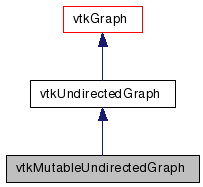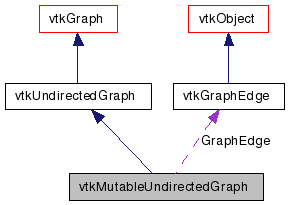# vtkMutableUndirectedGraph Class Reference

`#include <vtkMutableUndirectedGraph.h>`

Inheritance diagram for vtkMutableUndirectedGraph:[legend]
Collaboration diagram for vtkMutableUndirectedGraph:[legend]

## Detailed Description

An editable undirected graph.

vtkMutableUndirectedGraph is an undirected graph with additional functions for adding vertices and edges. ShallowCopy(), DeepCopy(), CheckedShallowCopy(), and CheckedDeepCopy() will succeed when the argument is a vtkUndirectedGraph or vtkMutableUndirectedGraph.

vtkUndirectedGraph vtkGraph
Tests:
vtkMutableUndirectedGraph (Tests)

Definition at line 44 of file vtkMutableUndirectedGraph.h.

## Public Types

typedef vtkUndirectedGraph Superclass

## Public Member Functions

virtual const char * GetClassName ()
virtual int IsA (const char *type)
void PrintSelf (ostream &os, vtkIndent indent)
virtual vtkIdType SetNumberOfVertices (vtkIdType numVerts)
vtkEdgeType AddEdge (vtkIdType u, vtkIdType v)
void LazyAddEdge (vtkIdType u, vtkIdType v)
void LazyAddEdge (vtkIdType u, vtkIdType v, vtkVariantArray *propertyArr)
void RemoveVertex (vtkIdType v)
void RemoveEdge (vtkIdType e)
void RemoveVertices (vtkIdTypeArray *arr)
void RemoveEdges (vtkIdTypeArray *arr)
vtkEdgeType AddEdge (vtkIdType u, vtkIdType v, vtkVariantArray *propertyArr)
vtkEdgeType AddEdge (const vtkVariant &u, vtkIdType v, vtkVariantArray *propertyArr=0)
vtkEdgeType AddEdge (vtkIdType u, const vtkVariant &v, vtkVariantArray *propertyArr=0)
vtkEdgeType AddEdge (const vtkVariant &u, const vtkVariant &v, vtkVariantArray *propertyArr=0)
void LazyAddEdge (const vtkVariant &u, vtkIdType v, vtkVariantArray *propertyArr=0)
void LazyAddEdge (vtkIdType u, const vtkVariant &v, vtkVariantArray *propertyArr=0)
void LazyAddEdge (const vtkVariant &u, const vtkVariant &v, vtkVariantArray *propertyArr=0)

## Static Public Member Functions

static vtkMutableUndirectedGraphNew ()
static int IsTypeOf (const char *type)
static vtkMutableUndirectedGraphSafeDownCast (vtkObject *o)

## Protected Member Functions

vtkMutableUndirectedGraph ()
~vtkMutableUndirectedGraph ()

## Protected Attributes

vtkGraphEdgeGraphEdge

## Member Typedef Documentation

Reimplemented from vtkUndirectedGraph.

Definition at line 48 of file vtkMutableUndirectedGraph.h.

## Constructor & Destructor Documentation

 vtkMutableUndirectedGraph::vtkMutableUndirectedGraph ( ) ` [protected]`

 vtkMutableUndirectedGraph::~vtkMutableUndirectedGraph ( ) ` [protected]`

## Member Function Documentation

 static vtkMutableUndirectedGraph* vtkMutableUndirectedGraph::New ( ) ` [static]`

Create an object with Debug turned off, modified time initialized to zero, and reference counting on.

Reimplemented from vtkUndirectedGraph.

 virtual const char* vtkMutableUndirectedGraph::GetClassName ( ) ` [virtual]`

Reimplemented from vtkUndirectedGraph.

 static int vtkMutableUndirectedGraph::IsTypeOf ( const char * name ) ` [static]`

Return 1 if this class type is the same type of (or a subclass of) the named class. Returns 0 otherwise. This method works in combination with vtkTypeMacro found in vtkSetGet.h.

Reimplemented from vtkUndirectedGraph.

 virtual int vtkMutableUndirectedGraph::IsA ( const char * name ) ` [virtual]`

Return 1 if this class is the same type of (or a subclass of) the named class. Returns 0 otherwise. This method works in combination with vtkTypeMacro found in vtkSetGet.h.

Reimplemented from vtkUndirectedGraph.

 static vtkMutableUndirectedGraph* vtkMutableUndirectedGraph::SafeDownCast ( vtkObject * o ) ` [static]`

Reimplemented from vtkUndirectedGraph.

 void vtkMutableUndirectedGraph::PrintSelf ( ostream & os, vtkIndent indent ) ` [virtual]`

Methods invoked by print to print information about the object including superclasses. Typically not called by the user (use Print() instead) but used in the hierarchical print process to combine the output of several classes.

Reimplemented from vtkUndirectedGraph.

 virtual vtkIdType vtkMutableUndirectedGraph::SetNumberOfVertices ( vtkIdType numVerts ) ` [virtual]`

Allocates space for the specified number of vertices in the graph's internal data structures. The previous number of vertices is returned on success and -1 is returned on failure. This has no effect on the number of vertex coordinate tuples or vertex attribute tuples allocated; you are responsible for guaranteeing these match. Also, this call is not implemented for distributed-memory graphs since the semantics are unclear; calling this function on a graph with a non-NULL DistributedGraphHelper will generate an error message, no allocation will be performed, and a value of -1 will be returned.

Adds a vertex to the graph and returns the index of the new vertex.

Note:
In a distributed graph (i.e. a graph whose DistributedHelper is non-null), this routine cannot be used to add a vertex if the vertices in the graph have pedigree IDs, because this routine will always add the vertex locally, which may conflict with the proper location of the vertex based on the distribution of the pedigree IDs.

 vtkIdType vtkMutableUndirectedGraph::AddVertex ( vtkVariantArray * propertyArr )

Adds a vertex to the graph with associated properties defined in `propertyArr` and returns the index of the new vertex. The number and order of values in `propertyArr` must match up with the arrays in the vertex data retrieved by GetVertexData(). If a vertex with the given pedigree ID already exists, its properties will be overwritten with the properties in `propertyArr` and the existing vertex index will be returned.

Note:
In a distributed graph (i.e. a graph whose DistributedHelper is non-null) the vertex added or found might not be local. In this case, AddVertex will wait until the vertex can be added or found remotely, so that the proper vertex index can be returned. If you don't actually need to use the vertex index, consider calling LazyAddVertex, which provides better performance by eliminating the delays associated with returning the vertex index.

 vtkIdType vtkMutableUndirectedGraph::AddVertex ( const vtkVariant & pedigreeId )

Adds a vertex with the given `pedigreeID` to the graph and returns the index of the new vertex. If a vertex with the given pedigree ID already exists, the existing vertex index will be returned.

Note:
In a distributed graph (i.e. a graph whose DistributedHelper is non-null) the vertex added or found might not be local. In this case, AddVertex will wait until the vertex can be added or found remotely, so that the proper vertex index can be returned. If you don't actually need to use the vertex index, consider calling LazyAddVertex, which provides better performance by eliminating the delays associated with returning the vertex index.

 vtkEdgeType vtkMutableUndirectedGraph::AddEdge ( vtkIdType u, vtkIdType v )

Adds an undirected edge from `u` to `v`, where `u` and `v` are vertex indices, and returns a `vtkEdgeType` structure describing that edge. `vtkEdgeType` contains fields for `Source` vertex index, `Target` vertex index, and edge index `Id`.

 vtkEdgeType vtkMutableUndirectedGraph::AddEdge ( vtkIdType u, vtkIdType v, vtkVariantArray * propertyArr )

Adds an undirected edge from `u` to `v`, where `u` and `v` are vertex indices, with associated properties defined in `propertyArr` and returns a `vtkEdgeType` structure describing that edge. The number and order of values in `propertyArr` must match up with the arrays in the edge data retrieved by GetEdgeData(). `vtkEdgeType` contains fields for `Source` vertex index, `Target` vertex index, and edge index `Id`.

 vtkEdgeType vtkMutableUndirectedGraph::AddEdge ( const vtkVariant & u, vtkIdType v, vtkVariantArray * propertyArr = `0` )

Adds an undirected edge from `u` to `v`, where `u` is a vertex pedigree ID and `v` is a vertex index, and returns a `vtkEdgeType` structure describing that edge. The number and order of values in the optional parameter `propertyArr` must match up with the arrays in the edge data retrieved by GetEdgeData(). `vtkEdgeType` contains fields for `Source` vertex index, `Target` vertex index, and edge index `Id`.

 vtkEdgeType vtkMutableUndirectedGraph::AddEdge ( vtkIdType u, const vtkVariant & v, vtkVariantArray * propertyArr = `0` )

Adds a directed edge from `u` to `v`, where `u` is a vertex index and `v` is a vertex pedigree ID, and returns a `vtkEdgeType` structure describing that edge. The number and order of values in the optional parameter `propertyArr` must match up with the arrays in the edge data retrieved by GetEdgeData(). `vtkEdgeType` contains fields for `Source` vertex index, `Target` vertex index, and edge index `Id`.

 vtkEdgeType vtkMutableUndirectedGraph::AddEdge ( const vtkVariant & u, const vtkVariant & v, vtkVariantArray * propertyArr = `0` )

Adds a directed edge from `u` to `v`, where `u` and `v` are vertex pedigree IDs, and returns a `vtkEdgeType` structure describing that edge. The number and order of values in the optional parameter `propertyArr` must match up with the arrays in the edge data retrieved by GetEdgeData(). `vtkEdgeType` contains fields for `Source` vertex index, `Target` vertex index, and edge index `Id`.

Adds a vertex to the graph. This method is lazily evaluated for distributed graphs (i.e. graphs whose DistributedHelper is non-null) the next time Synchronize is called on the helper.

 void vtkMutableUndirectedGraph::LazyAddVertex ( vtkVariantArray * propertyArr )

Adds a vertex to the graph with associated properties defined in `propertyArr`. The number and order of values in `propertyArr` must match up with the arrays in the vertex data retrieved by GetVertexData(). If a vertex with the given pedigree ID already exists, its properties will be overwritten with the properties in `propertyArr`. This method is lazily evaluated for distributed graphs (i.e. graphs whose DistributedHelper is non-null) the next time Synchronize is called on the helper.

 void vtkMutableUndirectedGraph::LazyAddVertex ( const vtkVariant & pedigreeId )

Adds a vertex with the given `pedigreeID` to the graph. If a vertex with the given pedigree ID already exists, no operation is performed. This method is lazily evaluated for distributed graphs (i.e. graphs whose DistributedHelper is non-null) the next time Synchronize is called on the helper.

 void vtkMutableUndirectedGraph::LazyAddEdge ( vtkIdType u, vtkIdType v )

Adds an undirected edge from `u` to `v`, where `u` and `v` are vertex indices. This method is lazily evaluated for distributed graphs (i.e. graphs whose DistributedHelper is non-null) the next time Synchronize is called on the helper.

 void vtkMutableUndirectedGraph::LazyAddEdge ( vtkIdType u, vtkIdType v, vtkVariantArray * propertyArr )

Adds an undirected edge from `u` to `v`, where `u` and `v` are vertex indices. The number and order of values in `propertyArr` must match up with the arrays in the edge data retrieved by GetEdgeData(). This method is lazily evaluated for distributed graphs (i.e. graphs whose DistributedHelper is non-null) the next time Synchronize is called on the helper.

 void vtkMutableUndirectedGraph::LazyAddEdge ( const vtkVariant & u, vtkIdType v, vtkVariantArray * propertyArr = `0` )

Adds an undirected edge from `u` to `v`, where `u` is a vertex pedigree ID and `v` is a vertex index. The number and order of values in the optional parameter `propertyArr` must match up with the arrays in the edge data retrieved by GetEdgeData(). This method is lazily evaluated for distributed graphs (i.e. graphs whose DistributedHelper is non-null) the next time Synchronize is called on the helper.

 void vtkMutableUndirectedGraph::LazyAddEdge ( vtkIdType u, const vtkVariant & v, vtkVariantArray * propertyArr = `0` )

Adds an undirected edge from `u` to `v`, where `u` is a vertex index and `v` is a vertex pedigree ID. The number and order of values in the optional parameter `propertyArr` must match up with the arrays in the edge data retrieved by GetEdgeData(). This method is lazily evaluated for distributed graphs (i.e. graphs whose DistributedHelper is non-null) the next time Synchronize is called on the helper.

 void vtkMutableUndirectedGraph::LazyAddEdge ( const vtkVariant & u, const vtkVariant & v, vtkVariantArray * propertyArr = `0` )

Adds an undirected edge from `u` to `v`, where `u` and `v` are vertex pedigree IDs. The number and order of values in the optional parameter `propertyArr` must match up with the arrays in the edge data retrieved by GetEdgeData(). This method is lazily evaluated for distributed graphs (i.e. graphs whose DistributedHelper is non-null) the next time Synchronize is called on the helper.

 vtkGraphEdge* vtkMutableUndirectedGraph::AddGraphEdge ( vtkIdType u, vtkIdType v )

Variant of AddEdge() that returns a heavyweight `vtkGraphEdge` object. The graph owns the reference of the edge and will replace its contents on the next call to AddGraphEdge().

Note:
This is a less efficient method for use with wrappers. In C++ you should use the faster AddEdge().

 void vtkMutableUndirectedGraph::RemoveVertex ( vtkIdType v )

Removes the vertex from the graph along with any connected edges. Note: This invalidates the last vertex index, which is reassigned to v.

 void vtkMutableUndirectedGraph::RemoveEdge ( vtkIdType e )

Removes the edge from the graph. Note: This invalidates the last edge index, which is reassigned to e.

 void vtkMutableUndirectedGraph::RemoveVertices ( vtkIdTypeArray * arr )

Removes a collection of vertices from the graph along with any connected edges.

 void vtkMutableUndirectedGraph::RemoveEdges ( vtkIdTypeArray * arr )

Removes a collection of edges from the graph.

## Member Data Documentation

 vtkGraphEdge* vtkMutableUndirectedGraph::GraphEdge` [protected]`

Graph edge that is reused of AddGraphEdge calls.

Definition at line 254 of file vtkMutableUndirectedGraph.h.

The documentation for this class was generated from the following file:

Generated on Wed Aug 24 11:49:26 2011 for VTK by1.5.6